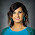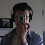## 3.13.2014

### Cheat Sheets vs. Formula Sheet vs. Nothing

What are your opinions on letting students use cheat sheets (of any form) or a formula sheet on a test?

I can see two sides. One, if a student uses a formula sheet (especially one used on an state exam or whatever) then it helps them get used to using it and it only gives them a formula. Using a cheat sheet can give students an example to look at it, which means they are possibly just changing numbers and plugging in without any true understanding of the concept. But then again, you could ask harder questions, such as application so that the formula is only a small part of what you want students to do.

The other side, giving students nothing, means you are assessing both memorization and application of the formula or skill.

Which do you prefer? Does it help or hurt students to use a cheat sheet or formula sheet?

Again, I can see two sides. One, if you're thinking about college, it probably hurts them because not many professors allow that but then again, some do. Two, if you're thinking about real life, if I'm being assessed on the job, I'm going to look up whatever information I need. It seems that in real life, performance matters more than memorization. That's why we have Google, right?

In addition, I seem to remember a lot of standardized tests that give the formula in the problem...again supporting the idea that application and performance are most important.

I guess it's just hard when you think about giving a test that requires several different formulas. If a student can't remember the formula, they have no chance at applying it. If they at least have a formula, they can attempt to apply it.

If using a cheat sheet, is there any merit to the idea that writing out worked examples helps with retention?

1.This comment has been removed by the author.

2.When teaching the quadratic formula, a colleague of mine would have them write down the formula and turn it in. When they turned it in, she would hand them the rest of the quiz on which the formula was written for their reference. So, she would give them some credit for knowing the formula but then let the rest of the focus be on application. I thought it was a decent compromise.

1.I wonder what she would do for a test that had multiple formulas?

3.I do different things depending on where I am in a lesson and what topic I'm assessing.

- If I know that a particular formula or trig identity will be available on the actual state or AP exam, then I will include it on every test and quiz that may involve that formula. I don't see much value in memorizing, say, angle sum or double angle identities in an introductory trig class. More importantly, I don't think a "Why do we have to memorize this when it's given to us on the state exam reference sheet?" argument is one where I want to spend my credibility and conviction currency with my students.

- When doing initial assessments of new topics, particularly when asking students to do something by a specific method (solve a quadratic equation by using the 'quadratic formula' instead of completing the square or factoring, for example), I will print the formula on the first couple of quizzes. I will also tell them directly that (a) this formula will not be printed on later tests and the state exam and (b) this is only one way to address a problem like this and they should think about which methods they prefer and which methods are better for various types of problems.

Like you said, not giving the formula in early assessments means you will be assessing memorization in addition to their ability to use the formula. Personally, I'd rather start out by seeing if they can implement the formula before worrying about them memorizing something.

As far as cheat sheets go, if you restrict them to the space they're allowed to use (e.g. give them an index card and say that they may only bring notes they can fit on that card), you might force them to think about the most important things to know and make them review everything in the process.

I don't know about writing out worked examples, but I do know that writing out formulas at the start of every homework problem I did in high school math and science courses resulted in me memorizing a lot of formulas without really trying.

1.Interesting, I kind of go the opposite way. I don't give them the formula on a quiz because the quiz is immediately after we've learned it and focused on that one skill.

When it comes time for a test, other concepts are put together and then there is more to memorize. That's when it seems most beneficial to me to give them a formula.

4.In New York State, students are given all of the sum/difference/double angle/half angle formulas on the Algebra 2//Trig Regents exam. So when I gave a recent quiz on the topic, I left all of the formulas on the board. What I found was that if students understood the topic, they were able to appropriately and correctly use the formulas, but if they didn't, the work was still a mystery to them. The formulas won't tell them what the correct reference angle measurements are, or what signs the trig functions should have in each quadrant. And they won't tell them how to add/subtract/multiply/divide fractions.

I know when the students are in Pre-Calculus, not only will they need to know the formulas from memory, but they will also need to understand how they are derived (we only dipped our toes into that pool during this unit). So I know that the time will come when they won't be able to use this support, but for the time being, I am only assessing their understanding of the underlying concepts and how to use them.

There are other formulas which I think students should know without a 'cheat sheet' - like how to to find slope, or the Pythagorean Theorem. These represent much bigger ideas in math. But then again, without true comprehension, students won't be able to use these formulas well either.